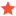Question regrading SolveIKYotamishJunior MemberPosts: 6 Threads: 4 Joined: Jan 2019 Reputation: 0 02-19-2019, 03:08 PM Hi, I'm receiving an Euler angles vector from another source, and want to move my Kuka arm to its location in joint space. I'm trying to use inverse kinematics to determine the arm joint configuration from the vector I'm receiving. However, moving in joint space to the IK result puts the arm in a different location than the position. I'm guessing it's because my arm reference frame is different than the main reference frame, and I'm doing something wrong while transforming between the two reference frames. Long story short, I think I'm doing something wrong in passing between two reference frames. Could you please help? what I need is something like this: ref1 = main_ref_frame ref2 = arm_base_ref_frame vec1 = (x,y,z,o1,o2,o3)  #vector representation in ref1 vec2 = ?????                  #vector representation in ref2 Thanks! Find ReplyAlbertModeratorPosts: 915 Threads: 1 Joined: Apr 2018 Reputation: 45 02-25-2019, 04:41 PM (This post was last modified: 07-07-2020, 04:25 PM by Albert.) You should use SolveIK to calculate the inverse kinematics. This will give you joint values given Cartesian and Euler angle information (for example, KUKA's XYZABC). SolveIK requires the pose of the robot flange to be provided with respect to the robot base. So you should do something like this: Code:```# enter the target information: The TCP (KUKA's \$TOOL) with respect to the reference (KUKA's \$BASE) target1_pose = KUKA_2_Pose([x,y,z,a,b,c]) base= KUKA_2_Pose([x,y,z,a,b,c]) tool = KUKA_2_Pose([x,y,z,a,b,c]) # Then you can calculate the robot pose: robot_pose = invH(base)*target1_pose*tool joints = SolveIK(robot_pose) # you can then use joints.list() to get the joint as a list of float``` The latest version also allows you to pass the tool pose and the reference pose like this: joints = SolveIK(robot_pose, tool, base) Find Reply